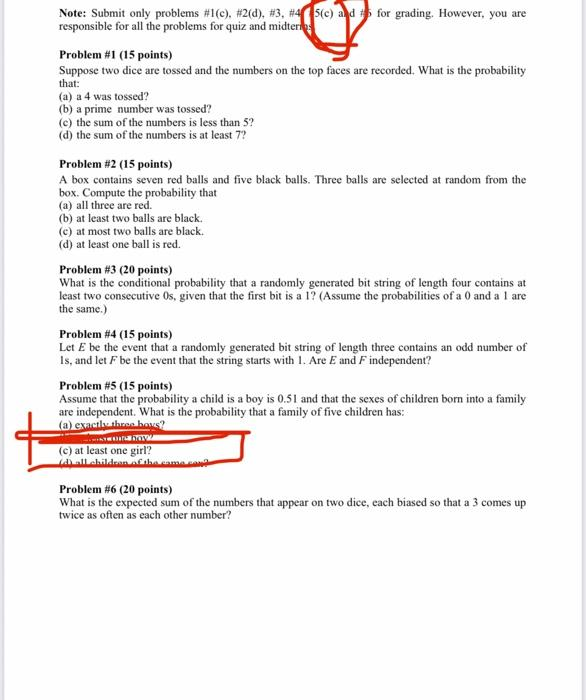# Question Note: Submit only problems #1(c). #2(d), #3, #45(e) and for grading. However, you are responsible for all the problems for quiz and midterste Problem #1 (15 points) Suppose two dice are tossed and the numbers on the top faces are recorded. What is the probability that: (a) a 4 was tossed? (b) a prime number was tossed? (c) the sum of the numbers is less than 5? (d) the sum of the numbers is at least 7? Problem #2 (15 points) A box contains seven red balls and five black balls. Three balls are selected at random from the box. Compute the probability that (a) all three are red. (b) at least two balls are black. (e) at most two balls are black (d) at least one ball is red. Problem #3 (20 points) What is the conditional probability that a randomly generated bit string of length four contains at least two consecutive Os, given that the first bit is a 1? (Assume the probabilities of a 0 and a 1 are the same.) Problem #4 (15 points) Let E be the event that a randomly generated bit string of length three contains an odd number of Is, and let F be the event that the string starts with 1. Are E and Findependent? Problem #5 (15 points) Assume that the probability a child is a boy is 0.51 and that the sexes of children born into a family are independent. What is the probability that a family of five children has: (a) exactly three boys? NOVI (e) at least one girl? سسسسسسسسلسعالمانيا للسي Problem #6 (20 points) What is the expected sum of the numbers that appear on two dice, cach biased so that a 3 comes up twice as often as each other number?OV4QFT The Asker · Computer ScienceTranscribed Image Text: Note: Submit only problems #1(c). #2(d), #3, #45(e) and for grading. However, you are responsible for all the problems for quiz and midterste Problem #1 (15 points) Suppose two dice are tossed and the numbers on the top faces are recorded. What is the probability that: (a) a 4 was tossed? (b) a prime number was tossed? (c) the sum of the numbers is less than 5? (d) the sum of the numbers is at least 7? Problem #2 (15 points) A box contains seven red balls and five black balls. Three balls are selected at random from the box. Compute the probability that (a) all three are red. (b) at least two balls are black. (e) at most two balls are black (d) at least one ball is red. Problem #3 (20 points) What is the conditional probability that a randomly generated bit string of length four contains at least two consecutive Os, given that the first bit is a 1? (Assume the probabilities of a 0 and a 1 are the same.) Problem #4 (15 points) Let E be the event that a randomly generated bit string of length three contains an odd number of Is, and let F be the event that the string starts with 1. Are E and Findependent? Problem #5 (15 points) Assume that the probability a child is a boy is 0.51 and that the sexes of children born into a family are independent. What is the probability that a family of five children has: (a) exactly three boys? NOVI (e) at least one girl? سسسسسسسسلسعالمانيا للسي Problem #6 (20 points) What is the expected sum of the numbers that appear on two dice, cach biased so that a 3 comes up twice as often as each other number?
More
Transcribed Image Text: Note: Submit only problems #1(c). #2(d), #3, #45(e) and for grading. However, you are responsible for all the problems for quiz and midterste Problem #1 (15 points) Suppose two dice are tossed and the numbers on the top faces are recorded. What is the probability that: (a) a 4 was tossed? (b) a prime number was tossed? (c) the sum of the numbers is less than 5? (d) the sum of the numbers is at least 7? Problem #2 (15 points) A box contains seven red balls and five black balls. Three balls are selected at random from the box. Compute the probability that (a) all three are red. (b) at least two balls are black. (e) at most two balls are black (d) at least one ball is red. Problem #3 (20 points) What is the conditional probability that a randomly generated bit string of length four contains at least two consecutive Os, given that the first bit is a 1? (Assume the probabilities of a 0 and a 1 are the same.) Problem #4 (15 points) Let E be the event that a randomly generated bit string of length three contains an odd number of Is, and let F be the event that the string starts with 1. Are E and Findependent? Problem #5 (15 points) Assume that the probability a child is a boy is 0.51 and that the sexes of children born into a family are independent. What is the probability that a family of five children has: (a) exactly three boys? NOVI (e) at least one girl? سسسسسسسسلسعالمانيا للسي Problem #6 (20 points) What is the expected sum of the numbers that appear on two dice, cach biased so that a 3 comes up twice as often as each other number?